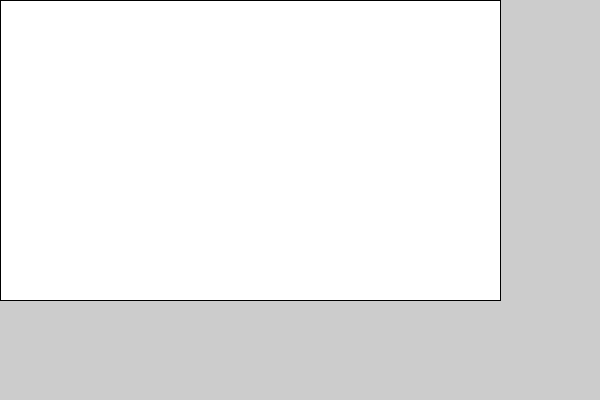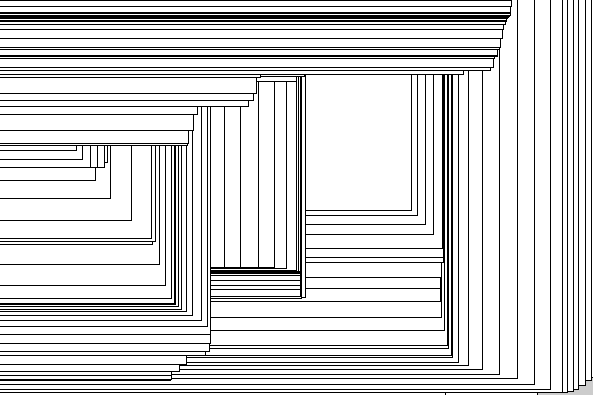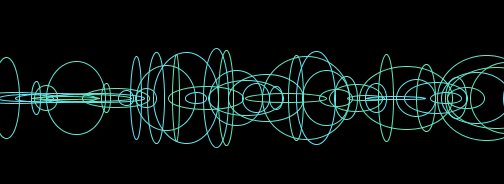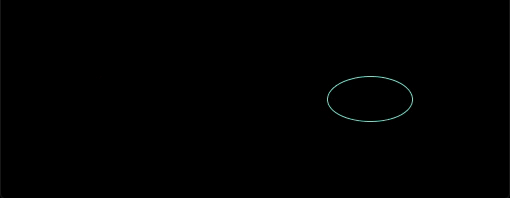# [Processing × Java] How to use variables1. Variable type
2. Example of a program using variables

# 0. What are variables

Variables are like ** boxes for data **.

#### `variable00.java`

``````
//Substitute 5 for the variable a.
int a = 5;
//Substitute 10 for the variable b.
int b = 10;

//a +b Display the result.
println(a + b);
``````
``````15
``````

Caution: When you use a variable in Java, you need to specify ** what kind of variable it is **. NG　:　`a = 5` OK　:　`int　a = 5`

Types include int for integers, float for floating point numbers, String for strings, and color for colors. Reference article: Java reference understood in the figure

Point: In the program, the equal sign (=) indicates ** substitution **. It's easy to understand if you think of it as a ** arrow pointing to the left **.

`int　a ⬅︎ 5`

# 1. Variable type

Variables include ** Built-in variables ** and ** User-defined variables **.

#### `variable01.java`

``````
size(600,400);
rect(0,0,width-100,height-100);

``````Point　:　Built-in variables Variables prepared in advance. width (= screen width), height (= screen height), mouseX (= mouse X coordinate), mouseY (= mouse Y coordinate) ... etc.

in this case, `width = 600` `height = 400` Can be used without having to define it yourself. lucky!

Point　:　User-defined variables Variables that you define and use. How to use: ① ** Declaration ** → ② ** Initial setting ** → ③ ** Use **

How to use (example) ① ** Declaration ** (I'm thinking of using such a variable!) `int a;` ② ** Initial setting ** (This variable should be set to this value!) `a = 10;` ③ ** Use ** (Then I will use it ...!) `println(a);`

• Declaration and initial setting can be done at the same time (Example) `int a = 5;`

# 2. Example of a program using variables

#### `variable02.java`

``````
//It is executed only once when the program starts.
void setup(){
//Determine the size of the screen
size(600,400);
}

//infinite loop
void draw(){
//The area of the rectangle changes depending on the location of the mouse.
rect(0,0,mouseX,mouseY);
}
``````Point　:　 setup () repeats the process in {} only once. (Something like initial settings) draw () repeats the process in {} infinitely. (infinite loop)

rect (X coordinate at the upper left corner of the rectangle, Y coordinate at the upper left corner of the rectangle, horizontal side length, vertical side length);

#### `variable03.java`

``````
//Repeat only once at the beginning
void setup(){
//Determine the size of the screen
size(510,510);
}
//infinite loop
void draw(){
//Do not draw the edge
noStroke();
//Decide how many colors to paint in the figure →(R,G,B)
fill(mouseX/2,mouseY/2,255);
//Determine the coordinates, width, and height of the center of the circle.
ellipse(mouseX,mouseY,30,30);
}
``````#### `variable04.java`

``````
//Define a float type variable xpos.
//A variable that represents the position of x.
float xpos = 0;

void setup(){
size(510,200);
background(0);
}

void draw(){
//Do not fill the inside of the circle.
noFill();
stroke(100,230,random(180,255));
//The x coordinate of the circle is determined by xpos.
//The size of the circle should be random.
ellipse(xpos,height/2,random(100),random(100));
//Increase xpos by 10.
xpos += 10;

}

``````#### `variable04.java`

``````
//Define a float type variable xpos.
//A variable that represents the position of x.
float xpos = 0;

void setup(){
size(510,200);
}

void draw(){
//Try repainting the background each time you loop.
background(0);
//Do not fill the inside of the circle.
noFill();
stroke(100,230,random(180,255));
//The x coordinate of the circle is determined by xpos.
//The size of the circle should be random.
ellipse(xpos,height/2,random(100),random(100));
//Increase xpos by 10.
xpos += 10;

//When the circle pops out of the screen, make it come out from the left again.
if(xpos > width){
xpos = 0;
}
}
``````# Finally

Thank you for reading. We appreciate your opinions and suggestions in order to make the article even better.